# Assignment 4

## COMP102/112 2022 Tri 1: Assignment 4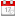Due 7 Apr 10am

#### Goals

This assignment will give you practice at writing programs that:
• use conditionals
• use for loop (foreach loops and for loops)
• have methods that return values

#### Preparation

Download the zip file for assignment 4 and extract it to the `COMP-102-112-2022T1-Assig4` folder in your home folder. It should contain templates for the Java program you are to complete.

Read through the exercises and run the demo so that you know what you need to do or view the demo videos

Later when the model answer to assignment 3 becomes available, make sure you understand all the components of the programs.

Also, go over the code examples from the lectures that used for loops.

#### To Submit

• Your version of `TemperatureAnalyser.java`
• Your version of `PatternsDrawer.java`

#### Summary

• Temperature Analyser: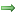Write a program to analyse and plot a series of temperature levels.
• Repetitive Patterns:Write a program to draw several different repetitive patterns

### Program 1: Temperature Analyser

Construct a `TemperatureAnalyser` program with several methods for analysing a list of readings of temperature levels from an experiment over the course of a day.

There are several things about the temperature levels that a user may be interested in:
• The average temperature level.
• How the temperatures rose and fell.
• The maximum and the minimum temperature levels during the day.

The `analyse()` method reads a sequence of levels from the user, using the `UI.askNumbers()` method. The sequence of levels is terminated by the word "done". `analyse()` then calls 4 methods which each do one part of the analysis.

You can run the `TemperatureAnalyser` demo program in the demonstrations folder to see what the methods should do. The demo has two buttons to help you see what needs to be done for the Core and for the Completion. You can also view the demo video.

##### Core:
• Complete the `printAverage` method so that it computes and prints out the average of the temperature levels.
• Complete the `plotLevels` method so that it plots the bars in the graphics pane.

You may assume that all the temperature levels are positive. For the core, it doesn't matter if a bar goes over the top of the window.

Note: If you select "Set Input" on the UI frame's MENU then choose the file `temperatures.txt`, the UI will automatically input the data from that file. This may help with testing your program.

##### Completion:

• Extend the `plotLevels` method so that it also draws a horizontal line for the x-axis (or baseline). You do not need any labels on the axis.
• Extend the `plotLevels` method so that it handles temperature levels over the maximum (400) by plotting a bar of the maximum size and putting an asterisk ("*") above it to show that it has been cut off.
• Complete the `maximumOfList` method so that it finds and returns the maximum levels in the list.
• Complete the `minimumOfList` method so that it finds and returns the minimum levels in the list.

##### Challenge:

• Extend the `plotLevels` method to draw both an x-axis and a y-axis, and put labels on them. You need to make design decision, e.g. for the y-axis have labels at roughly every 50 pixels, for x-axis make meaningful labels, such as if there are 30 bars number every third, or if there are 500 only do every 50.
• Extend the `plotLevels` method to draw negative temperature levels correctly
• Extend the `plotLevels` method to use the maximum temperature level to scale the y-axis so that the maximum level would reach just to the top of the graph.
• Extend the `plotLevels` method to use the number of temperature levels to scale the x-axis so that all the bars fit in the window.
• Extend the `plotLevels` method to compute the standard deviation (sd) and show the mean and 1sd on the plot
• Add a `medianOfList` that will compute and return the median value in the list.

##### Hints:
The average of a set of numbers is the sum of the numbers, divided by the number of levels.

To find the minimum and the maximum, your loop will need to keep track of the smallest and largest levels seen so far.

You may want to use the values `Double.MAX_VALUE` and `Double.MIN_VALUE` which are the largest and smallest numbers that Java recognises.

When designing and constructing a program, it is usually a good idea to get the basic part of the program working before adding the extra features. It would be a good idea to first make the `analyse` method just read the levels and print out the average, and let the other methods print a message. Once you have that working, you can extend the program to also deal with the plotting, the minimum, and the maximum.

### Program 2: Repetitive Patterns

You can run the `TemperatureAnalyserPatternsDrawer` demo program or view the video to see what the methods should do.

There are many patterns made of repetitive elements used in a wide variety of applications, ranging from board games to vinyl floor coverings. Complete the methods in the `PatternsDrawer` program for drawing a range of these patterns. Each method requires using loops. The position and size of the pattern are specified by the three constants `PATTERN_LEFT`, `PATTERN_TOP`, and `PATTERN_SIZE`. Each method starts by asking the user for a number: the size. The loops should use this number!

#### Core

• Row Pattern: a row of filled rectangles, alternating between the colours blue and orange.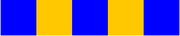• Checkers board - the standard board for chess and checkers/draughts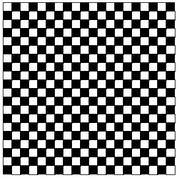This needs two nested loops but must sometimes draw a black square and sometimes an white square.
Hint: if the row and column are both even, or both odd, then it is white. (Remember %, the 'remainder' operator!)

#### Completion

• Illusion - a pattern that makes dark circles appear in the intersections when you look at it.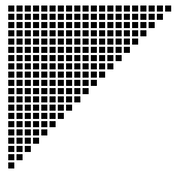Complete the `drawTriGrid` method: this also requires two nested loops, but each row must have a different number of squares.

#### Challenge

• Concentric circles - a grid of concentric circles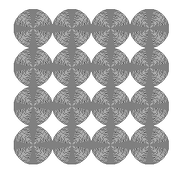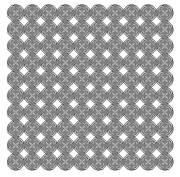The concentric circles should be separated by one pixel. The larger the cells of the grid (ie, the fewer number of cells) the more circles there will be. The first example had 4 rows and columns, while the second had 10 rows and columns.

### To go further

It is important to understand how Java works, as well as writing programs in Java.
1. `printAverage` computed and printed the average, but `minimumOfList` just computed and returned the minimum - it didn't print it out. Which design is better and why? What are the advantages of making a method do multiple things, and what are the advantages of making a method do just one thing?
2. Several of the methods used nested loops (a for loop inside a for loop). Could you have written the methods using just one for loop? Would it be better? Why not?